# SQLite

iOS 系統支援很常見的資料庫 SQLite ，這是一個輕量的關聯式資料庫管理系統( Relational Database Management System ，縮寫為 RDBMS )，所有的資料內容其實就是一個檔案，而且絕大部分的 SQL 指令都可以使用。

### 加入 SQLite

#### 加入 sqlite 函式庫

▼ 預設的應用程式沒有引入 sqlite 函式庫，所以需要手動加入，先找到`TARGETS > ExSQLite > General > Linked Frameworks and Libraries`，並按下加號`+`，如下圖：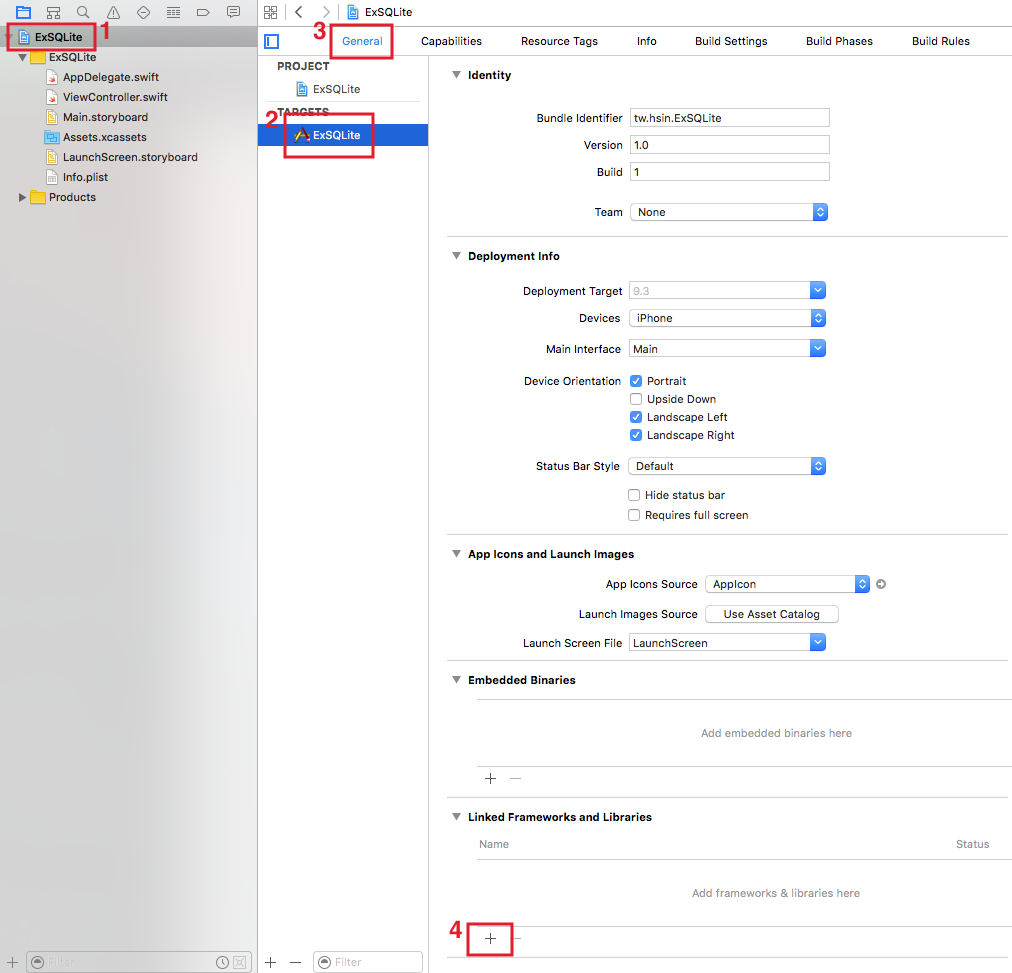▼ 這邊會列出來所有可以加入的函式庫，所以填入`sqlite`來過濾，最後會出現兩個類似的函式庫，實際上兩個指的是一樣的東西，所以選一個加入就好，這邊選取`libsqlite3.tbd`，按下`Add`加入：▼ 如果有順利加入的話， sqlite 函式庫就會出現在列表中，如下圖：▼ 前面提到需要利用 Objective-C 來與 SQLite (也就是前一步驟加入的 sqlite 函式庫)連結，所以接著必須為應用程式加上一個 header 檔案以連結。先以新增檔案的方式加入，但請注意選擇檔案類型時，要選擇`iOS > Source > Header File`，如下圖：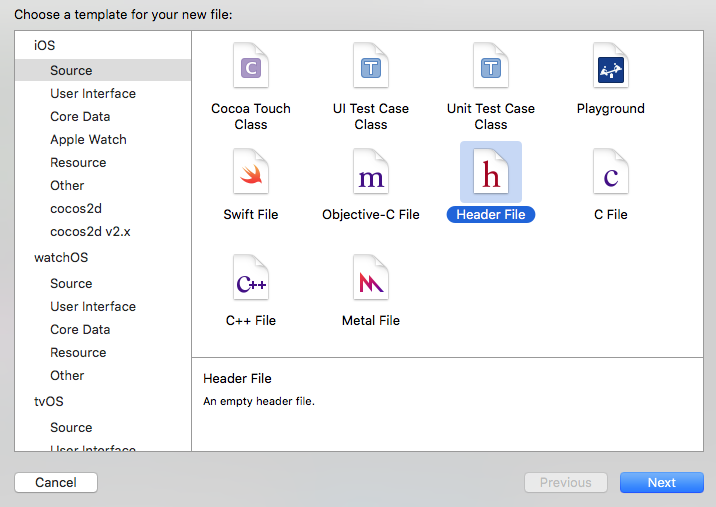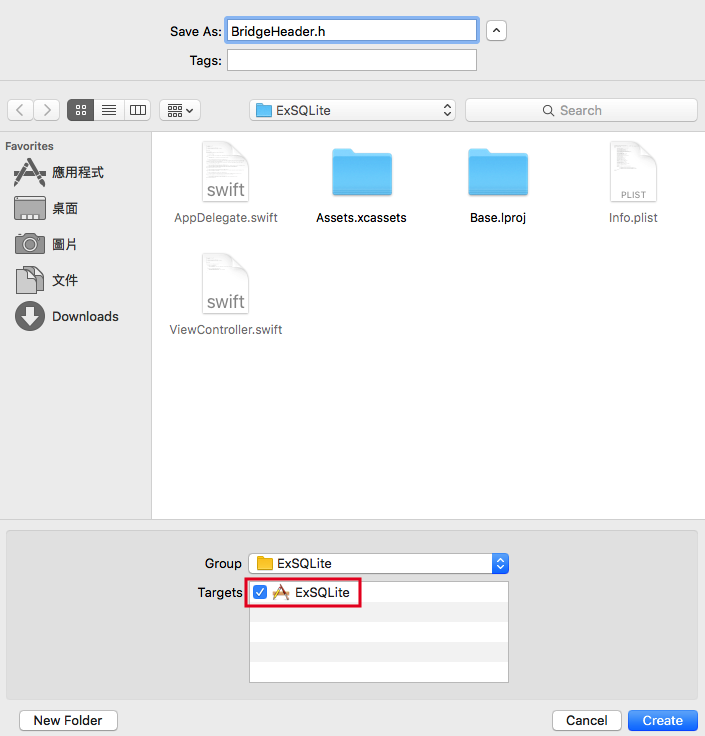``````#include "sqlite3.h"
``````

#### 與 Objective-C 連結

▼ 最後要將 Swift 與前一步驟設置好的 header 檔案連結起來，首先找到`TARGETS > ExSQLite > Build Settings > Objective-C Bridging Header`，這邊有很多東西可以設定，所以你可以在右上角的搜尋框輸入`bridg`來過濾，如下圖：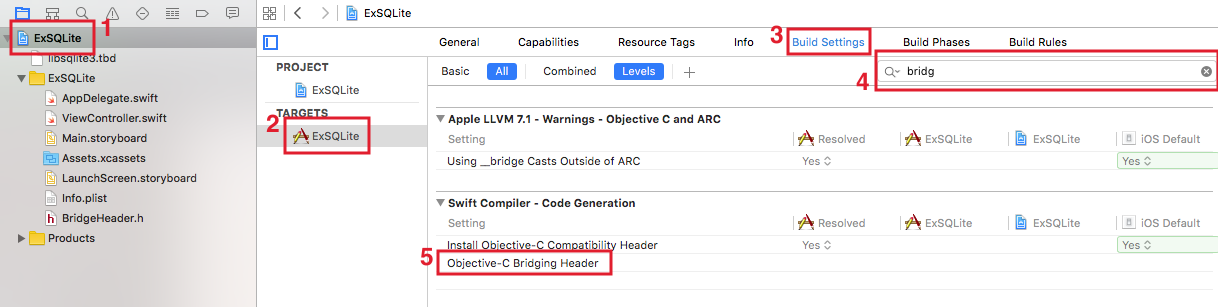▼ 接著對下圖標示`1`的地方點兩下，會彈出一個輸入框，填入 header 檔案的路徑與名稱(請記得路徑也要填)：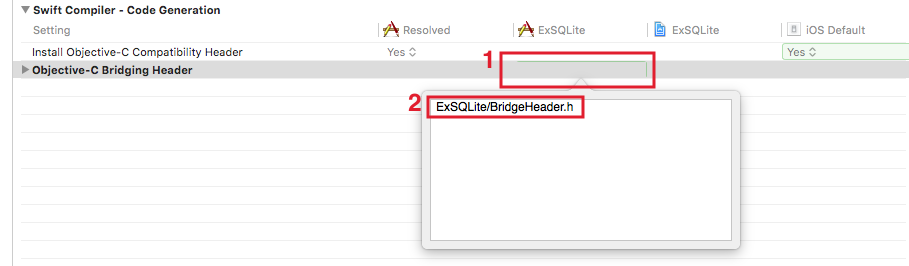▼ 如果順利加入的話，會顯示如下圖：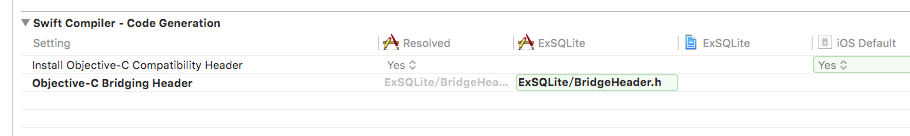### 使用 SQLite

``````var db :COpaquePointer = nil
``````

#### 資料庫檔案路徑

``````// 資料庫檔案的路徑
let urls = NSFileManager.defaultManager()
.URLsForDirectory(
.DocumentDirectory,
let sqlitePath = urls[urls.count-1].absoluteString
+ "sqlite3.db"
``````

#### 開啟資料庫連線

``````if sqlite3_open(sqlitePath, &db) == SQLITE_OK {
print("資料庫連線成功")
} else {
print("資料庫連線失敗")
}
``````

#### 建立資料表

``````let sql = "create table if not exists students "
+ "( id integer primary key autoincrement, "
+ "name text, height double)" as NSString

if sqlite3_exec(db, sql.UTF8String, nil, nil, nil)
== SQLITE_OK{
print("建立資料表成功")
}
``````

##### Hint
• 如果要查看模擬器的 SQLite 檔案，可以使用桌機的 Firefox 瀏覽器的一個套件 SQLite Manager ，它可以讓你檢視與編輯本機上的 SQLite 檔案。實際檔案路徑可藉由將前面提過的`sqlitePath`印出來取得。

#### 新增資料

``````var statement :COpaquePointer = nil
let sql = "insert into students "
+ "(name, height) "
+ "values ('小強', 178.2)" as NSString

if sqlite3_prepare_v2(
db, sql.UTF8String, -1, &statement, nil) == SQLITE_OK{
if sqlite3_step(statement) == SQLITE_DONE {
print("新增資料成功")
}
sqlite3_finalize(statement)
}
``````

`statement`要再當做`sqlite3_step()`函式的參數傳入，如果返回`SQLITE_DONE`，則是表示新增成功。

#### 讀取資料

``````var statement :COpaquePointer = nil
var sql = "select * from students"
sqlite3_prepare_v2(
db, (sql as NSString).UTF8String, -1, &statement, nil)

while sqlite3_step(statement) == SQLITE_ROW{
let id = sqlite3_column_int(statement, 0)
let name = String.fromCString(
UnsafePointer<CChar>(sqlite3_column_text(statement, 1)))
let height = sqlite3_column_double(statement, 2)
print("\(id). \(name!) 身高： \(height)")
}
sqlite3_finalize(statement)
``````

#### 更新資料

``````var statement :COpaquePointer = nil
var sql = "update students set name='小強' where id = 1"

if sqlite3_prepare_v2(
db, (sql as NSString).UTF8String, -1, &statement, nil)
== SQLITE_OK {
if sqlite3_step(statement) == SQLITE_DONE {
print("更新資料成功")
}
sqlite3_finalize(statement)
}
``````

#### 刪除資料

``````var statement :COpaquePointer = nil
var sql = "delete from students where id = 3"

if sqlite3_prepare_v2(
db, (sql as NSString).UTF8String, -1, &statement, nil)
== SQLITE_OK {
if sqlite3_step(statement) == SQLITE_DONE {
print("刪除資料成功")
}
sqlite3_finalize(statement)
}
``````

### 將 SQLite 功能獨立出來

`iOS > Source > Swift File`

``````class SQLiteConnect {

var db :COpaquePointer = nil
let sqlitePath :String

init?(path :String) {
sqlitePath = path
db = self.openDatabase(sqlitePath)

if db == nil {
return nil
}
}

// 連結資料庫 connect database
func openDatabase(path :String) -> COpaquePointer {
var connectdb: COpaquePointer = nil
if sqlite3_open(path, &connectdb) == SQLITE_OK {
print("Successfully opened database \(path)")
return connectdb
} else {
print("Unable to open database.")
return nil
}
}

// 建立資料表 create table
func createTable(
tableName :String, columnsInfo :[String]) -> Bool {
let sql = "create table if not exists \(tableName) "
+ "(\(columnsInfo.joinWithSeparator(",")))"
as NSString

if sqlite3_exec(
self.db, sql.UTF8String, nil, nil, nil) == SQLITE_OK{
return true
}

return false
}

// 新增資料
func insert(
tableName :String, rowInfo :[String:String]) -> Bool {
var statement :COpaquePointer = nil
let sql = "insert into \(tableName) "
+ "(\(rowInfo.keys.joinWithSeparator(","))) "
+ "values "
+"(\(rowInfo.values.joinWithSeparator(",")))"
as NSString

if sqlite3_prepare_v2(
self.db, sql.UTF8String, -1, &statement, nil)
== SQLITE_OK {
if sqlite3_step(statement) == SQLITE_DONE {
return true
}
sqlite3_finalize(statement)
}

return false
}

// 讀取資料
func fetch(
tableName :String, cond :String?, order :String?)
-> COpaquePointer {
var statement :COpaquePointer = nil
var sql = "select * from \(tableName)"
if let condition = cond {
sql += " where \(condition)"
}

if let orderBy = order {
sql += " order by \(orderBy)"
}

sqlite3_prepare_v2(
self.db, (sql as NSString).UTF8String, -1,
&statement, nil)

return statement
}

// 更新資料
func update(
tableName :String,
cond :String?, rowInfo :[String:String]) -> Bool {
var statement :COpaquePointer = nil
var sql = "update \(tableName) set "

// row info
var info :[String] = []
for (k, v) in rowInfo {
info.append("\(k) = \(v)")
}
sql += info.joinWithSeparator(",")

// condition
if let condition = cond {
sql += " where \(condition)"
}

if sqlite3_prepare_v2(
self.db, (sql as NSString).UTF8String, -1,
&statement, nil) == SQLITE_OK {
if sqlite3_step(statement) == SQLITE_DONE {
return true
}
sqlite3_finalize(statement)
}

return false

}

// 刪除資料
func delete(tableName :String, cond :String?) -> Bool {
var statement :COpaquePointer = nil
var sql = "delete from \(tableName)"

// condition
if let condition = cond {
sql += " where \(condition)"
}

if sqlite3_prepare_v2(
self.db, (sql as NSString).UTF8String, -1,
&statement, nil) == SQLITE_OK {
if sqlite3_step(statement) == SQLITE_DONE {
return true
}
sqlite3_finalize(statement)
}

return false
}

}
``````

``````// 資料庫檔案的路徑
let sqlitePath = NSHomeDirectory() + "/Documents/sqlite3.db"

// 印出儲存檔案的位置
print(sqlitePath)

// SQLite 資料庫
db = SQLiteConnect(path: sqlitePath)

if let mydb = db {

// create table
mydb.createTable("students", columnsInfo: [
"id integer primary key autoincrement",
"name text",
"height double"])

// insert
mydb.insert(
"students", rowInfo:
["name":"'大強'","height":"178.2"])

// select
let statement = mydb.fetch(
"students", cond: "1 == 1", order: nil)
while sqlite3_step(statement) == SQLITE_ROW{
let id = sqlite3_column_int(statement, 0)
let name =
String.fromCString(UnsafePointer<CChar>(
sqlite3_column_text(statement, 1)))
let height = sqlite3_column_double(statement, 2)
print("\(id). \(name!) 身高： \(height)")
}
sqlite3_finalize(statement)

// update
mydb.update(
"students",
cond: "id = 1",
rowInfo: ["name":"'小強'","height":"176.8"])

// delete
mydb.delete("students", cond: "id = 5")

}
``````